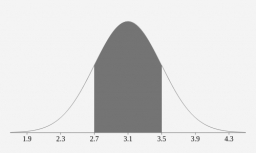# Two methods

A teacher wishes two methods of teaching verbs to his English class. He divided the class randomly into two samples of 14 students each. Using Method 1, the students learned an average of 43.8 with a standard deviation of 4.6, while the second group (Method 2) got an average of 38.6 with a standard deviation of 5. Method 1 is superior to method 2. Test using a 0.01 level of significance.

x =  0Did you find an error or inaccuracy? Feel free to write us. Thank you!

Tips for related online calculators
Looking for help with calculating arithmetic mean?
Looking for a statistical calculator?
Looking for a standard deviation calculator?

#### Grade of the word problem:

We encourage you to watch this tutorial video on this math problem: